Скачать презентацию Engineering Economics — EIT Review Cash Flow Evaluation

b0cd0d05a2ccbe19dde47d8a7eed6e36.ppt

• Количество слайдов: 47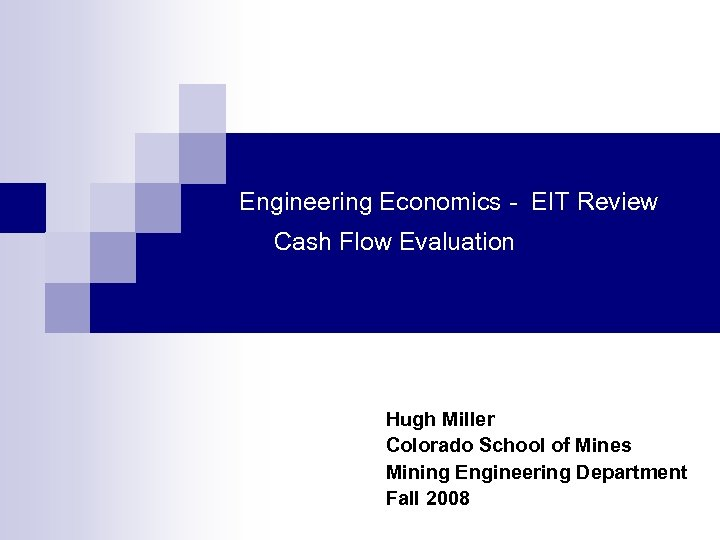Engineering Economics - EIT Review Cash Flow Evaluation Hugh Miller Colorado School of Mines Mining Engineering Department Fall 2008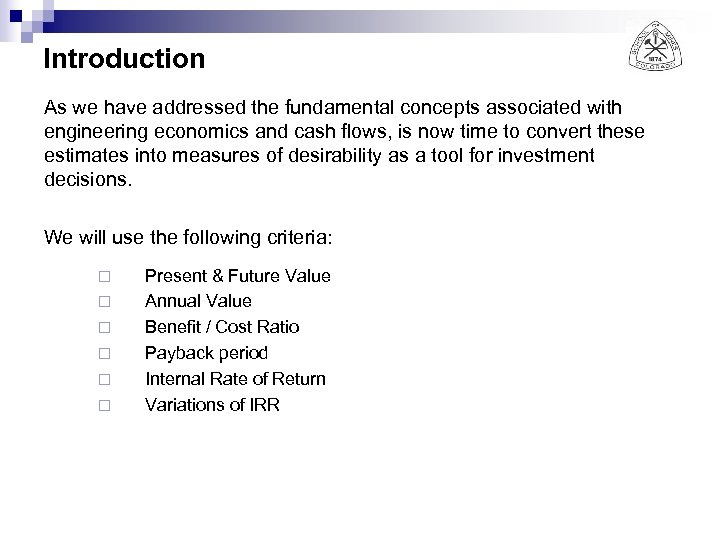Introduction As we have addressed the fundamental concepts associated with engineering economics and cash flows, is now time to convert these estimates into measures of desirability as a tool for investment decisions. We will use the following criteria: ¨ ¨ ¨ Present & Future Value Annual Value Benefit / Cost Ratio Payback period Internal Rate of Return Variations of IRR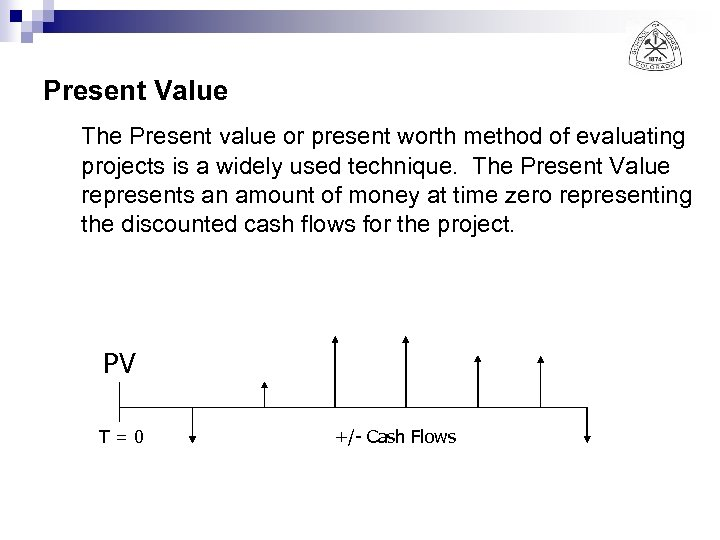Present Value The Present value or present worth method of evaluating projects is a widely used technique. The Present Value represents an amount of money at time zero representing the discounted cash flows for the project. PV T=0 +/- Cash Flows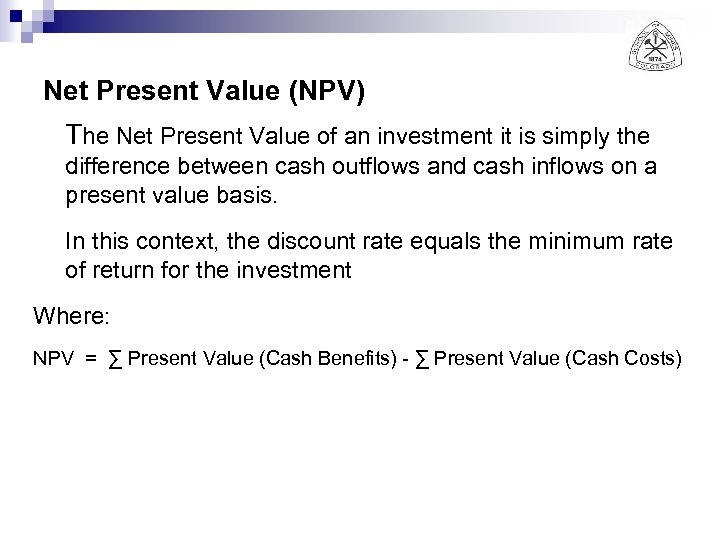Net Present Value (NPV) The Net Present Value of an investment it is simply the difference between cash outflows and cash inflows on a present value basis. In this context, the discount rate equals the minimum rate of return for the investment Where: NPV = ∑ Present Value (Cash Benefits) - ∑ Present Value (Cash Costs)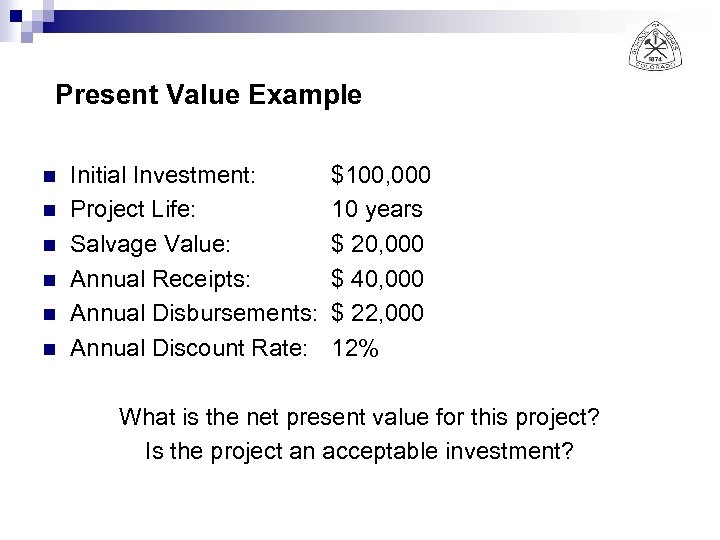Present Value Example n n n Initial Investment: Project Life: Salvage Value: Annual Receipts: Annual Disbursements: Annual Discount Rate: \$100, 000 10 years \$ 20, 000 \$ 40, 000 \$ 22, 000 12% What is the net present value for this project? Is the project an acceptable investment?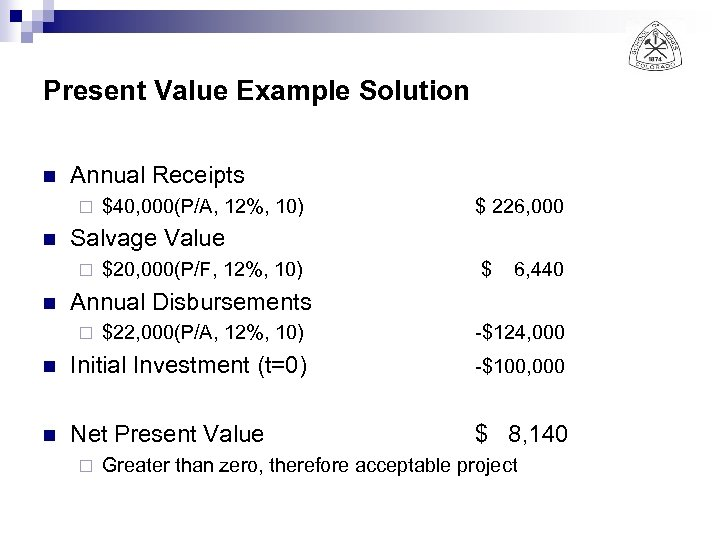Present Value Example Solution n Annual Receipts ¨ n \$ 226, 000 Salvage Value ¨ n \$40, 000(P/A, 12%, 10) \$20, 000(P/F, 12%, 10) \$ 6, 440 Annual Disbursements \$22, 000(P/A, 12%, 10) -\$124, 000 n Initial Investment (t=0) -\$100, 000 n Net Present Value \$ 8, 140 ¨ ¨ Greater than zero, therefore acceptable project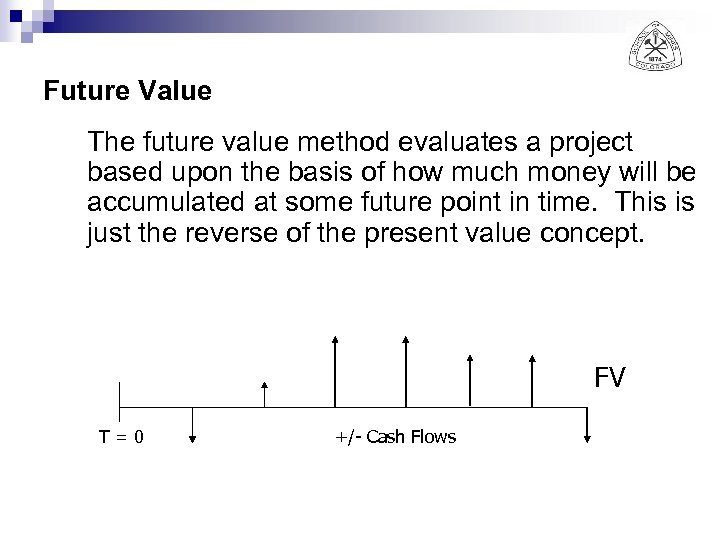Future Value The future value method evaluates a project based upon the basis of how much money will be accumulated at some future point in time. This is just the reverse of the present value concept. FV T=0 +/- Cash Flows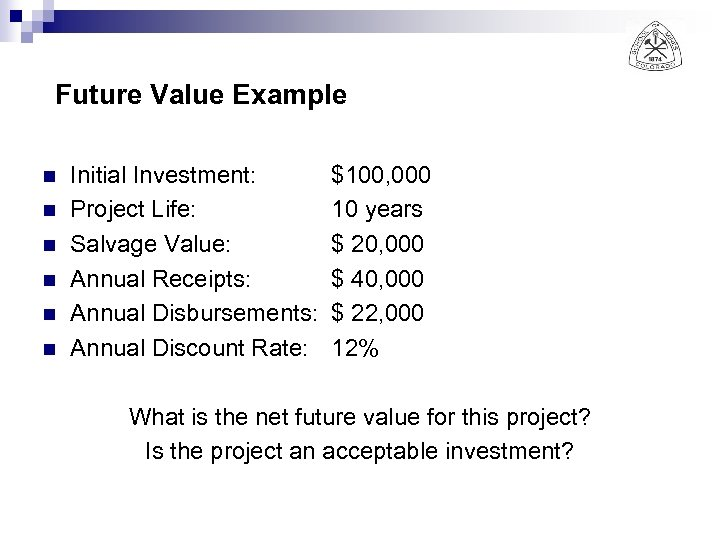Future Value Example n n n Initial Investment: Project Life: Salvage Value: Annual Receipts: Annual Disbursements: Annual Discount Rate: \$100, 000 10 years \$ 20, 000 \$ 40, 000 \$ 22, 000 12% What is the net future value for this project? Is the project an acceptable investment?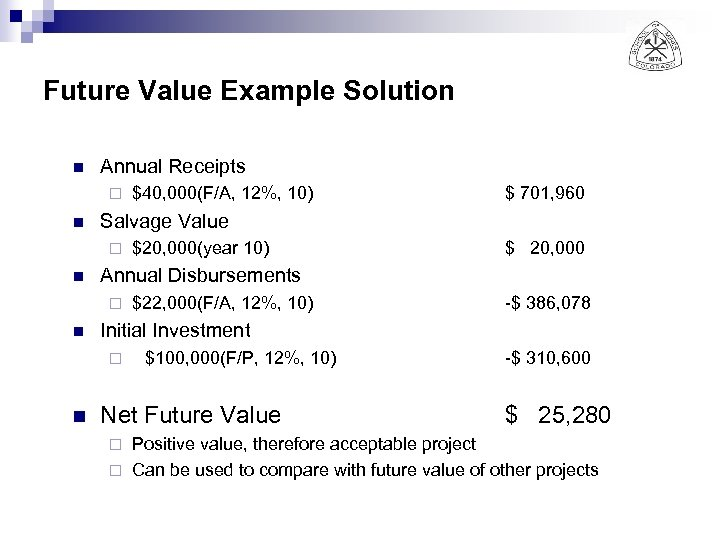Future Value Example Solution n Annual Receipts ¨ n \$ 20, 000 \$22, 000(F/A, 12%, 10) -\$ 386, 078 Initial Investment ¨ n \$20, 000(year 10) Annual Disbursements ¨ n \$ 701, 960 Salvage Value ¨ n \$40, 000(F/A, 12%, 10) \$100, 000(F/P, 12%, 10) Net Future Value -\$ 310, 600 \$ 25, 280 Positive value, therefore acceptable project ¨ Can be used to compare with future value of other projects ¨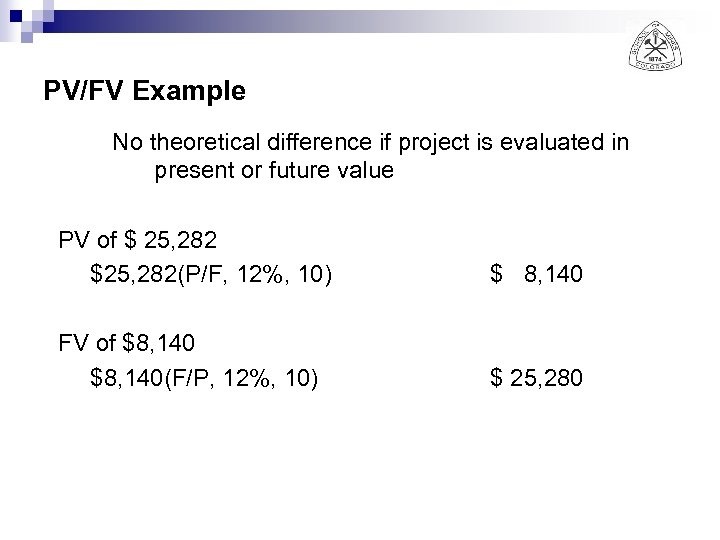PV/FV Example No theoretical difference if project is evaluated in present or future value PV of \$ 25, 282 \$25, 282(P/F, 12%, 10) \$ 8, 140 FV of \$8, 140(F/P, 12%, 10) \$ 25, 280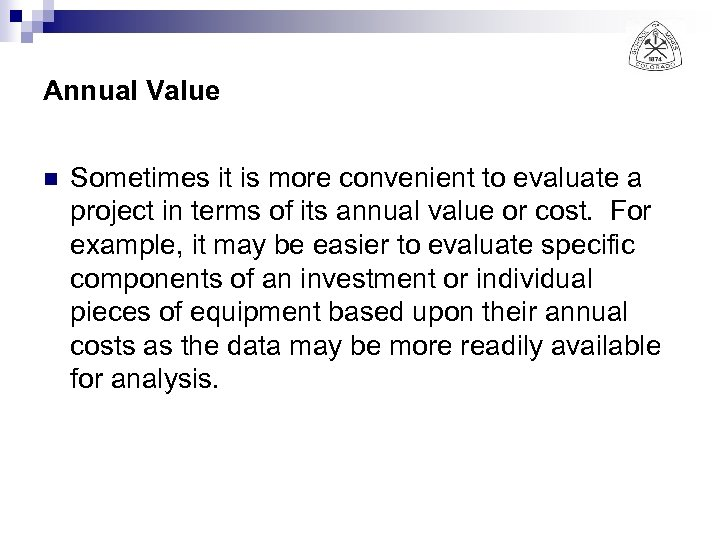Annual Value n Sometimes it is more convenient to evaluate a project in terms of its annual value or cost. For example, it may be easier to evaluate specific components of an investment or individual pieces of equipment based upon their annual costs as the data may be more readily available for analysis.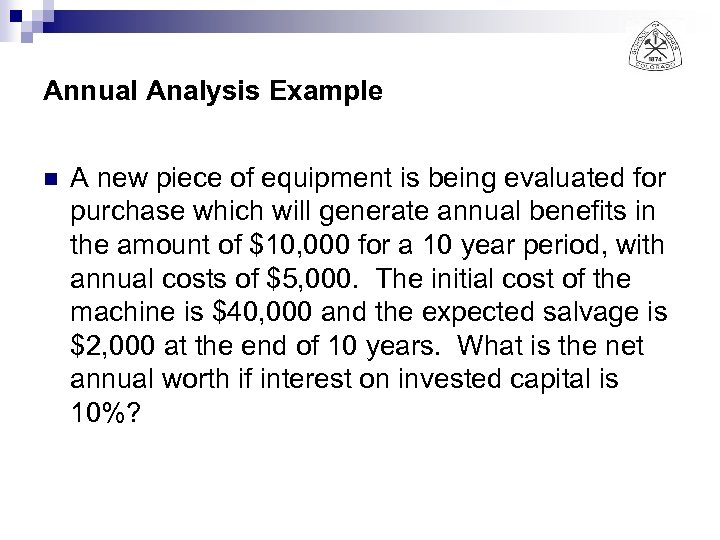Annual Analysis Example n A new piece of equipment is being evaluated for purchase which will generate annual benefits in the amount of \$10, 000 for a 10 year period, with annual costs of \$5, 000. The initial cost of the machine is \$40, 000 and the expected salvage is \$2, 000 at the end of 10 years. What is the net annual worth if interest on invested capital is 10%?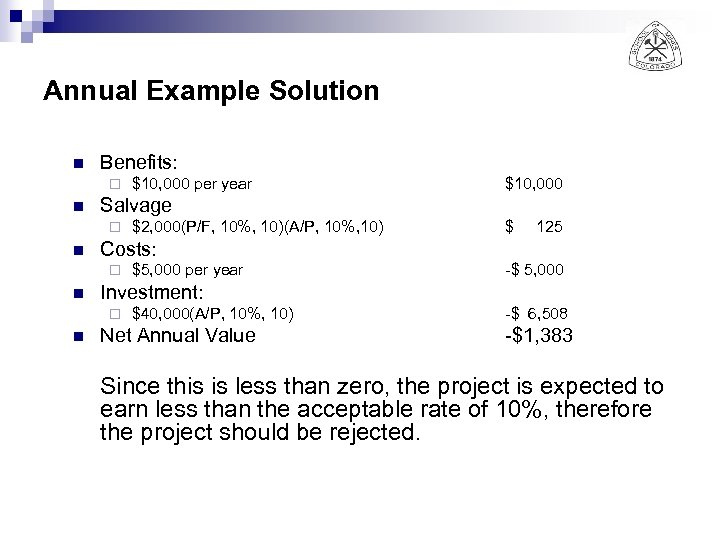Annual Example Solution n Benefits: ¨ n \$ 125 \$5, 000 per year -\$ 5, 000 Investment: ¨ n \$2, 000(P/F, 10%, 10)(A/P, 10%, 10) Costs: ¨ n \$10, 000 Salvage ¨ n \$10, 000 per year \$40, 000(A/P, 10%, 10) Net Annual Value -\$ 6, 508 -\$1, 383 Since this is less than zero, the project is expected to earn less than the acceptable rate of 10%, therefore the project should be rejected.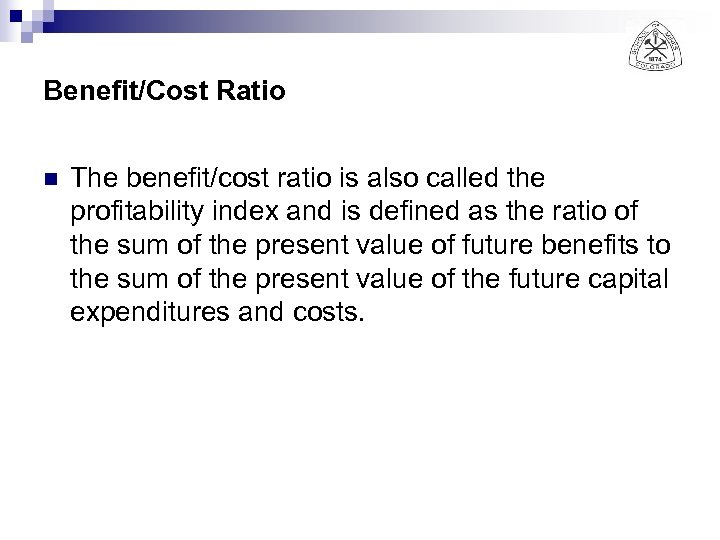Benefit/Cost Ratio n The benefit/cost ratio is also called the profitability index and is defined as the ratio of the sum of the present value of future benefits to the sum of the present value of the future capital expenditures and costs.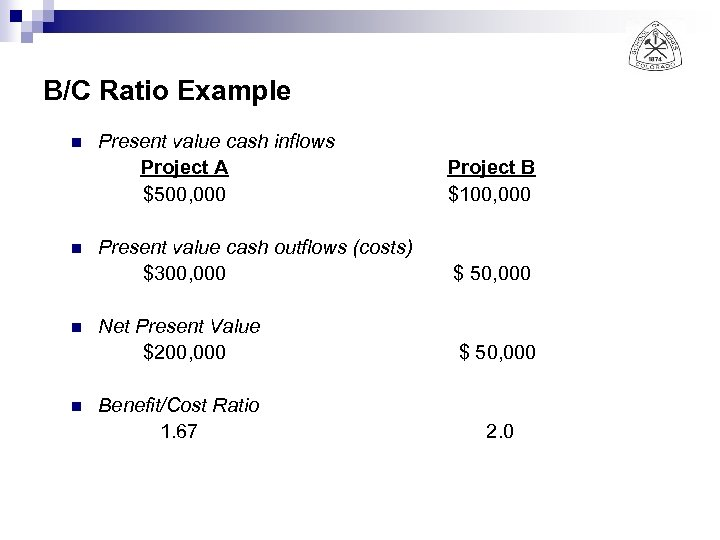B/C Ratio Example n n Present value cash inflows Project A \$500, 000 Project B \$100, 000 Present value cash outflows (costs) \$300, 000 \$ 50, 000 Net Present Value \$200, 000 \$ 50, 000 Benefit/Cost Ratio 1. 67 2. 0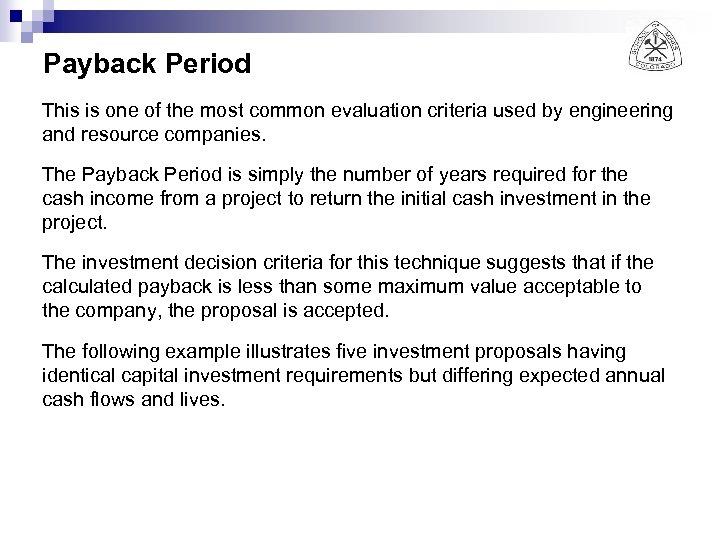Payback Period This is one of the most common evaluation criteria used by engineering and resource companies. The Payback Period is simply the number of years required for the cash income from a project to return the initial cash investment in the project. The investment decision criteria for this technique suggests that if the calculated payback is less than some maximum value acceptable to the company, the proposal is accepted. The following example illustrates five investment proposals having identical capital investment requirements but differing expected annual cash flows and lives.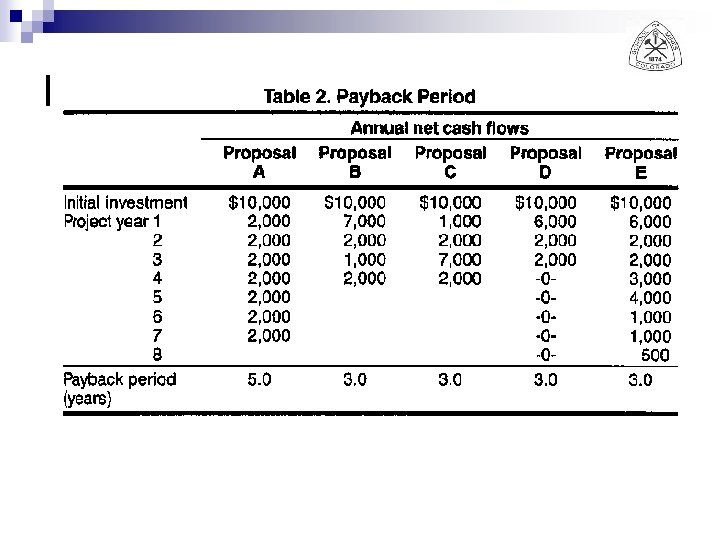Payback Period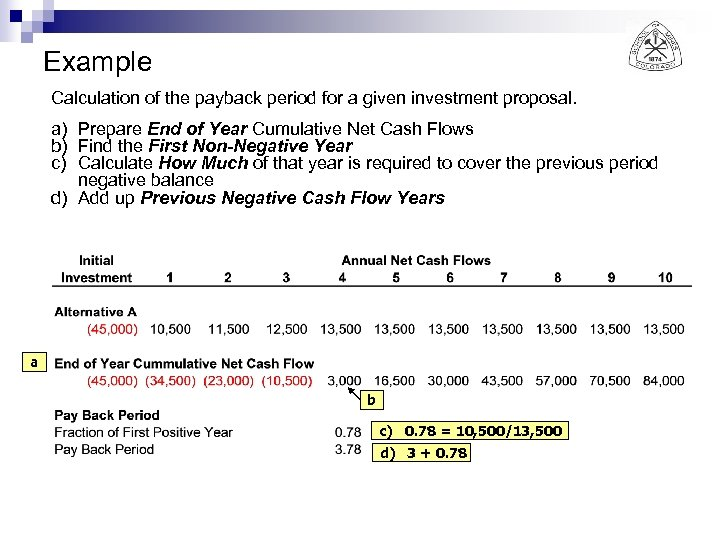Example Calculation of the payback period for a given investment proposal. a) Prepare End of Year Cumulative Net Cash Flows b) Find the First Non-Negative Year c) Calculate How Much of that year is required to cover the previous period negative balance d) Add up Previous Negative Cash Flow Years a b c) 0. 78 = 10, 500/13, 500 d) 3 + 0. 78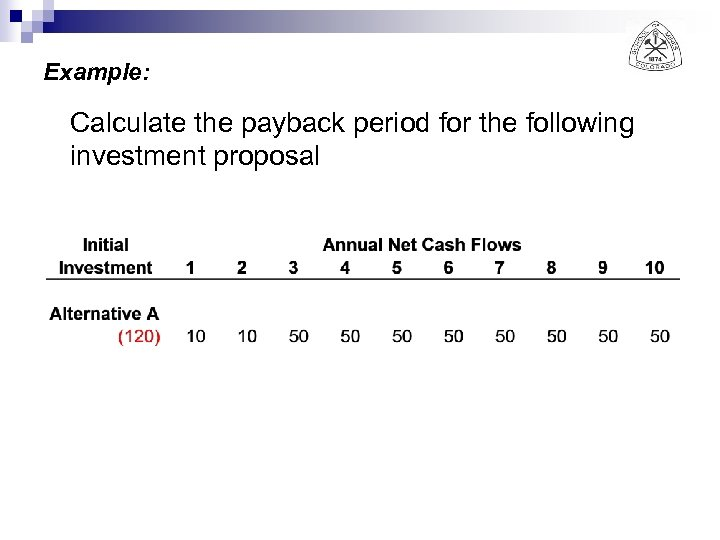Example: Calculate the payback period for the following investment proposal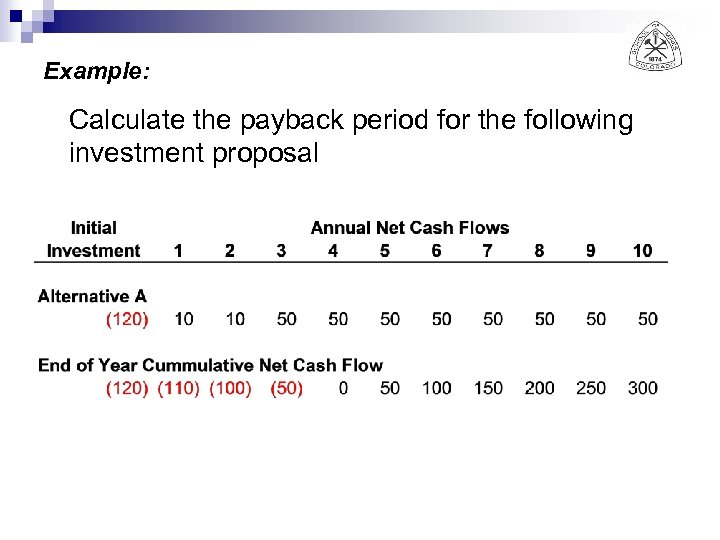Example: Calculate the payback period for the following investment proposal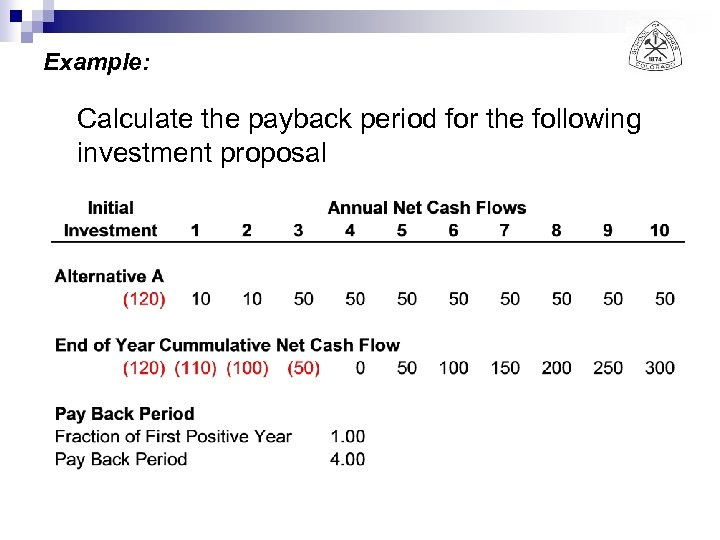Example: Calculate the payback period for the following investment proposal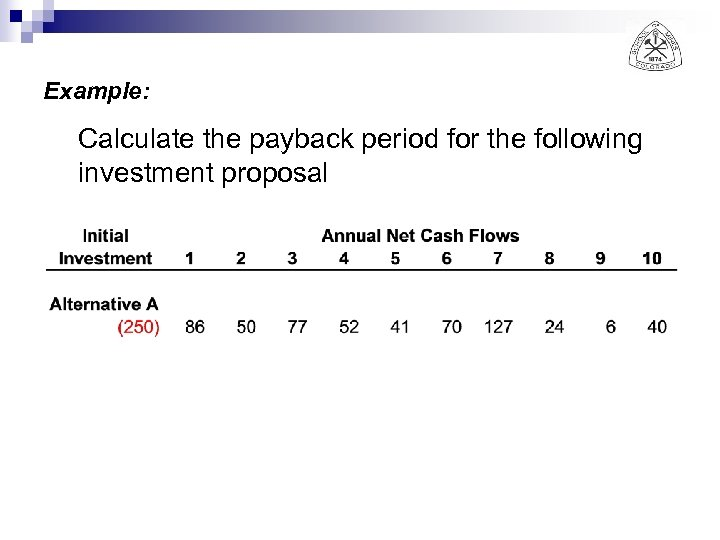Example: Calculate the payback period for the following investment proposal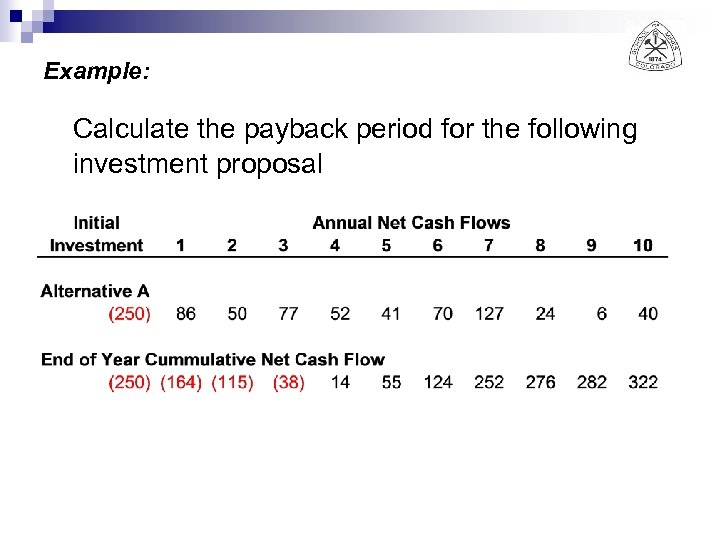Example: Calculate the payback period for the following investment proposal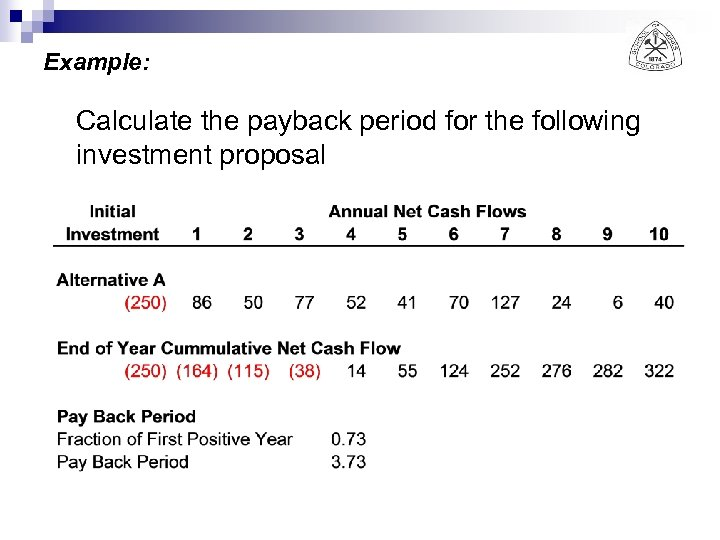Example: Calculate the payback period for the following investment proposal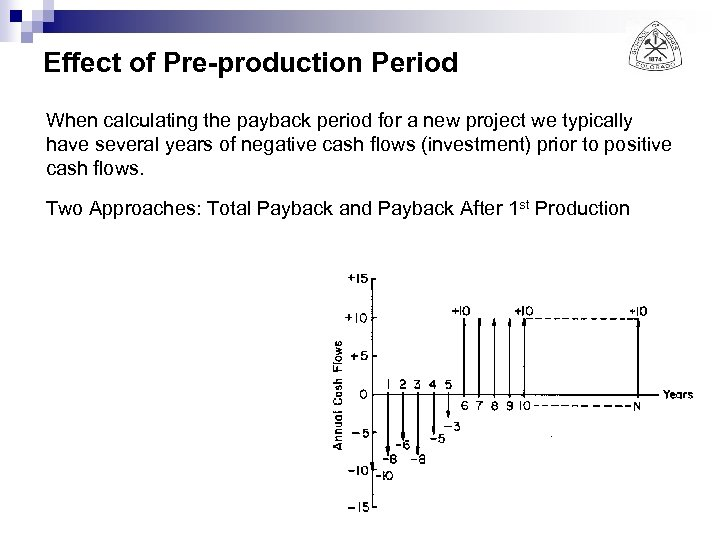Effect of Pre-production Period When calculating the payback period for a new project we typically have several years of negative cash flows (investment) prior to positive cash flows. Two Approaches: Total Payback and Payback After 1 st Production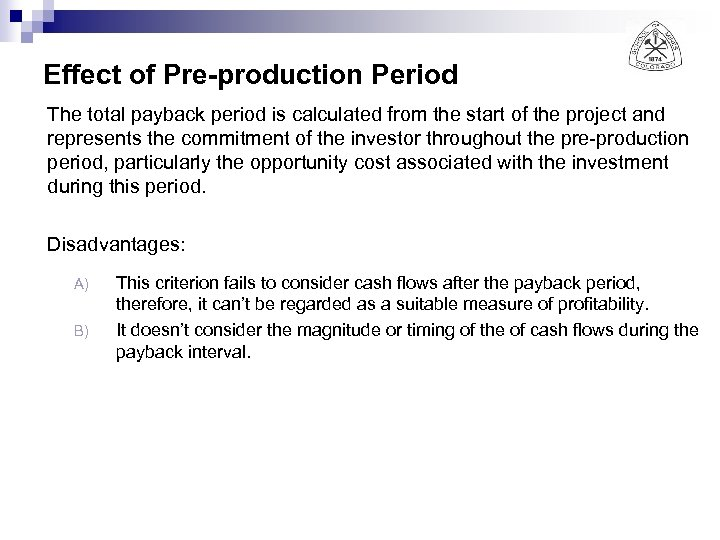Effect of Pre-production Period The total payback period is calculated from the start of the project and represents the commitment of the investor throughout the pre-production period, particularly the opportunity cost associated with the investment during this period. Disadvantages: A) B) This criterion fails to consider cash flows after the payback period, therefore, it can’t be regarded as a suitable measure of profitability. It doesn’t consider the magnitude or timing of the of cash flows during the payback interval.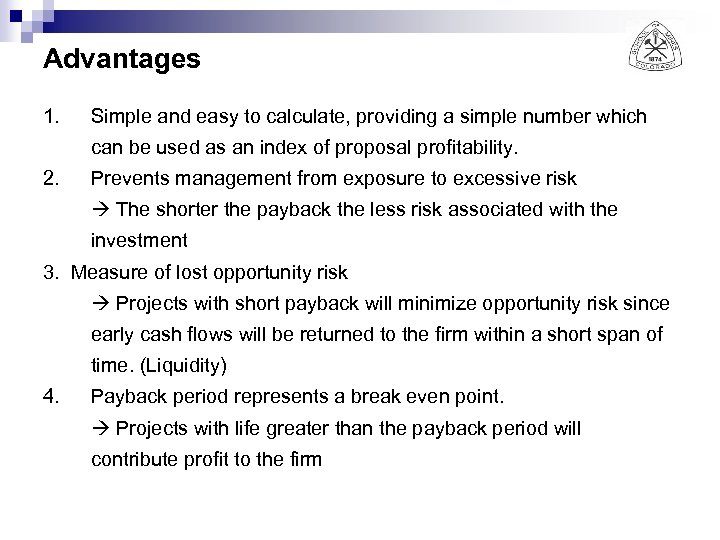Advantages 1. Simple and easy to calculate, providing a simple number which can be used as an index of proposal profitability. 2. Prevents management from exposure to excessive risk The shorter the payback the less risk associated with the investment 3. Measure of lost opportunity risk Projects with short payback will minimize opportunity risk since early cash flows will be returned to the firm within a short span of time. (Liquidity) 4. Payback period represents a break even point. Projects with life greater than the payback period will contribute profit to the firm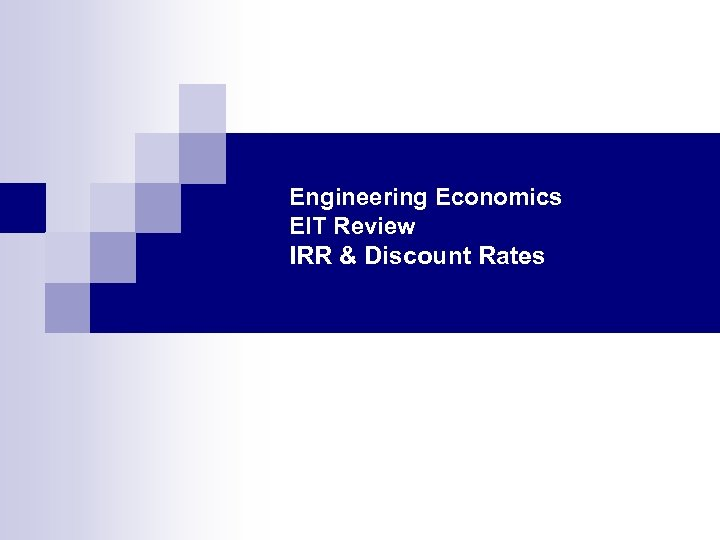Engineering Economics EIT Review IRR & Discount Rates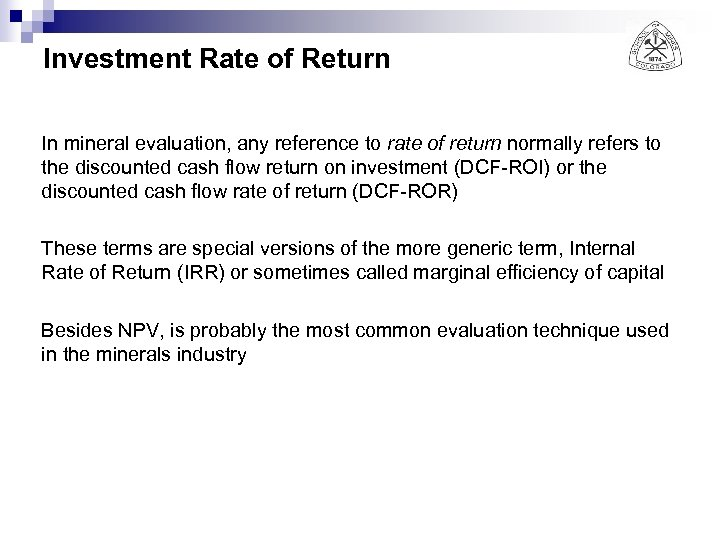Investment Rate of Return In mineral evaluation, any reference to rate of return normally refers to the discounted cash flow return on investment (DCF-ROI) or the discounted cash flow rate of return (DCF-ROR) These terms are special versions of the more generic term, Internal Rate of Return (IRR) or sometimes called marginal efficiency of capital Besides NPV, is probably the most common evaluation technique used in the minerals industry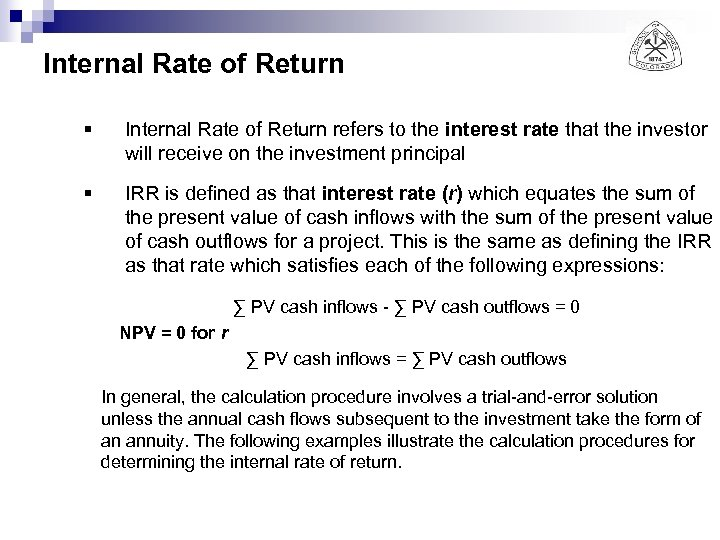Internal Rate of Return § Internal Rate of Return refers to the interest rate that the investor will receive on the investment principal § IRR is defined as that interest rate (r) which equates the sum of the present value of cash inflows with the sum of the present value of cash outflows for a project. This is the same as defining the IRR as that rate which satisfies each of the following expressions: ∑ PV cash inflows - ∑ PV cash outflows = 0 NPV = 0 for r ∑ PV cash inflows = ∑ PV cash outflows In general, the calculation procedure involves a trial-and-error solution unless the annual cash flows subsequent to the investment take the form of an annuity. The following examples illustrate the calculation procedures for determining the internal rate of return.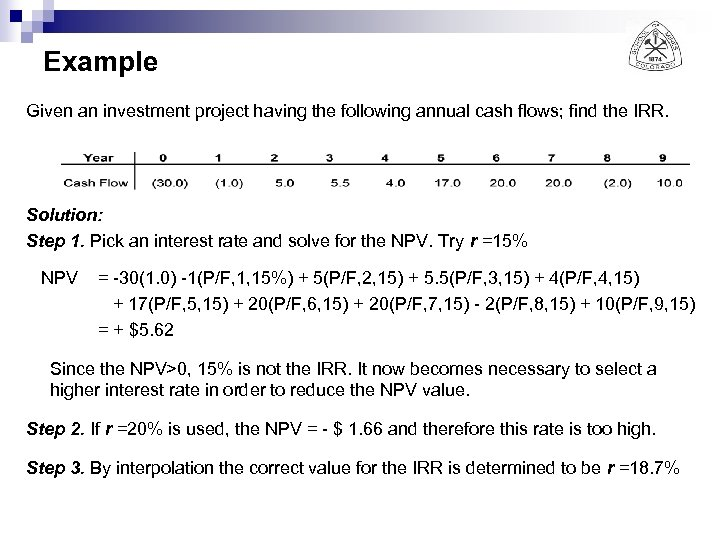Example Given an investment project having the following annual cash flows; find the IRR. Solution: Step 1. Pick an interest rate and solve for the NPV. Try r =15% NPV = -30(1. 0) -1(P/F, 1, 15%) + 5(P/F, 2, 15) + 5. 5(P/F, 3, 15) + 4(P/F, 4, 15) + 17(P/F, 5, 15) + 20(P/F, 6, 15) + 20(P/F, 7, 15) - 2(P/F, 8, 15) + 10(P/F, 9, 15) = + \$5. 62 Since the NPV>0, 15% is not the IRR. It now becomes necessary to select a higher interest rate in order to reduce the NPV value. Step 2. If r =20% is used, the NPV = - \$ 1. 66 and therefore this rate is too high. Step 3. By interpolation the correct value for the IRR is determined to be r =18. 7%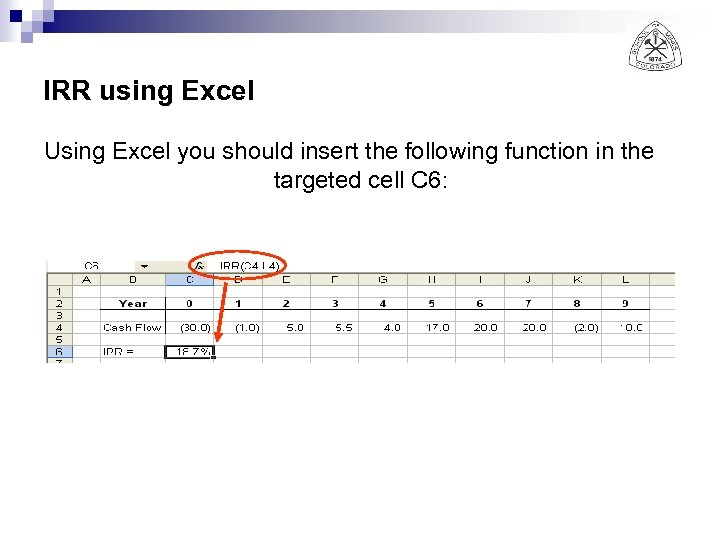IRR using Excel Using Excel you should insert the following function in the targeted cell C 6: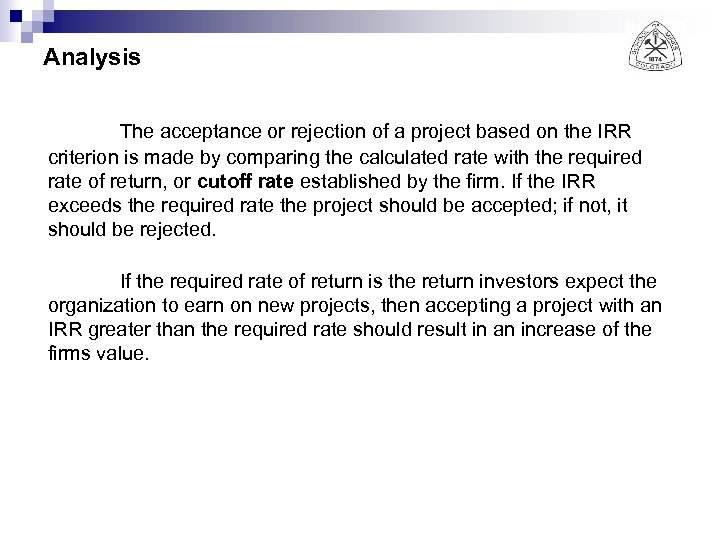Analysis The acceptance or rejection of a project based on the IRR criterion is made by comparing the calculated rate with the required rate of return, or cutoff rate established by the firm. If the IRR exceeds the required rate the project should be accepted; if not, it should be rejected. If the required rate of return is the return investors expect the organization to earn on new projects, then accepting a project with an IRR greater than the required rate should result in an increase of the firms value.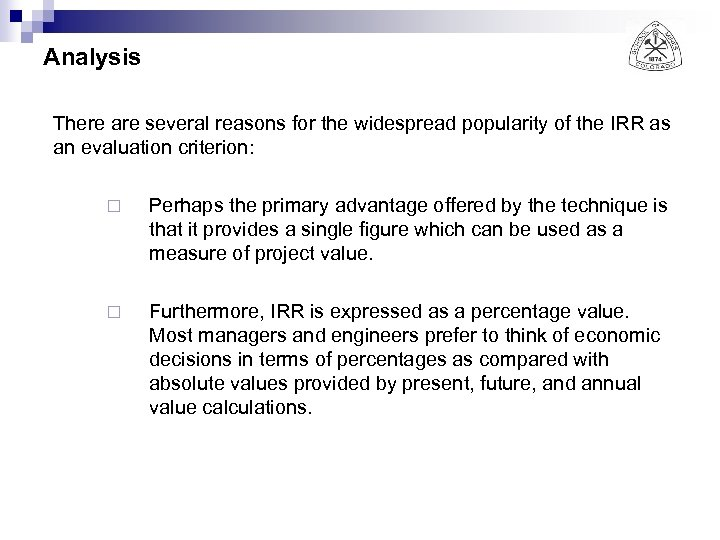Analysis There are several reasons for the widespread popularity of the IRR as an evaluation criterion: ¨ Perhaps the primary advantage offered by the technique is that it provides a single figure which can be used as a measure of project value. ¨ Furthermore, IRR is expressed as a percentage value. Most managers and engineers prefer to think of economic decisions in terms of percentages as compared with absolute values provided by present, future, and annual value calculations.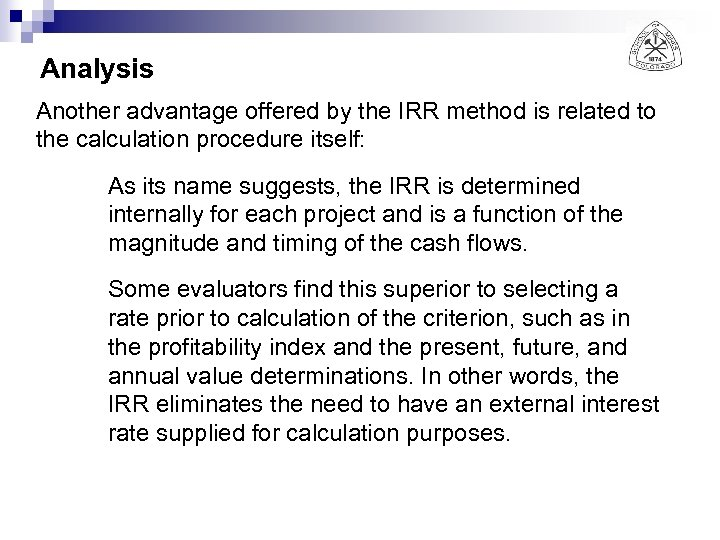Analysis Another advantage offered by the IRR method is related to the calculation procedure itself: As its name suggests, the IRR is determined internally for each project and is a function of the magnitude and timing of the cash flows. Some evaluators find this superior to selecting a rate prior to calculation of the criterion, such as in the profitability index and the present, future, and annual value determinations. In other words, the IRR eliminates the need to have an external interest rate supplied for calculation purposes.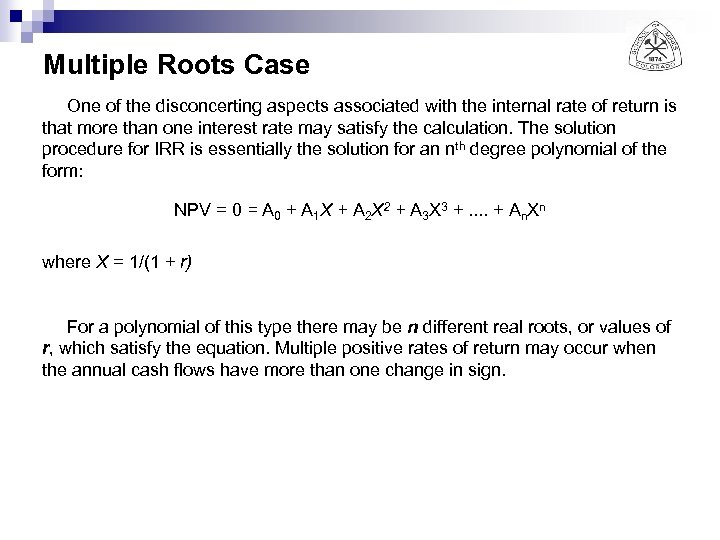Multiple Roots Case One of the disconcerting aspects associated with the internal rate of return is that more than one interest rate may satisfy the calculation. The solution procedure for IRR is essentially the solution for an nth degree polynomial of the form: NPV = 0 = A 0 + A 1 X + A 2 X 2 + A 3 X 3 +. . + An. Xn where X = 1/(1 + r) For a polynomial of this type there may be n different real roots, or values of r, which satisfy the equation. Multiple positive rates of return may occur when the annual cash flows have more than one change in sign.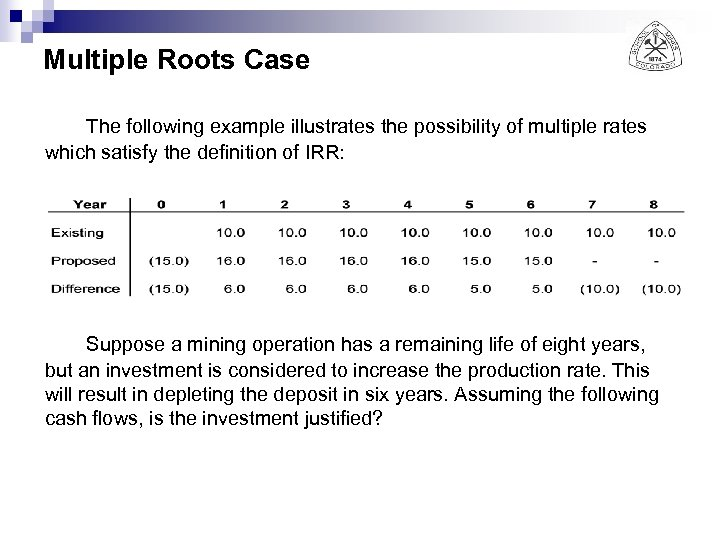Multiple Roots Case The following example illustrates the possibility of multiple rates which satisfy the definition of IRR: Suppose a mining operation has a remaining life of eight years, but an investment is considered to increase the production rate. This will result in depleting the deposit in six years. Assuming the following cash flows, is the investment justified?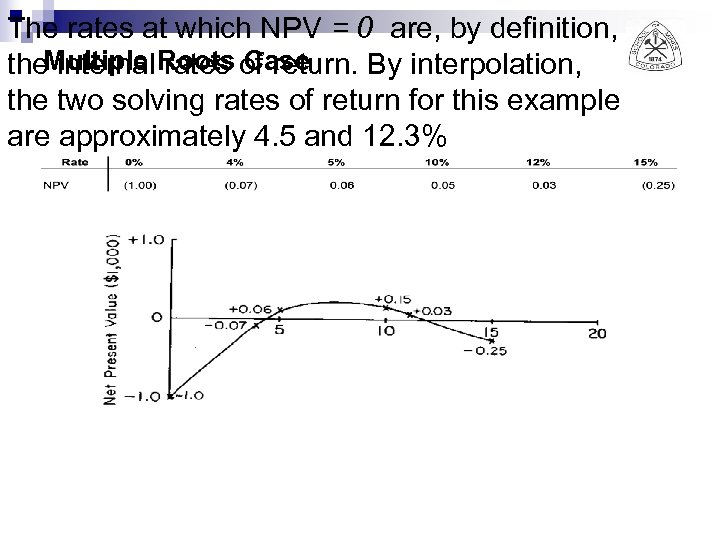The rates at which NPV = 0 are, by definition, the. Multiple Roots of return. By interpolation, internal rates Case the two solving rates of return for this example are approximately 4. 5 and 12. 3%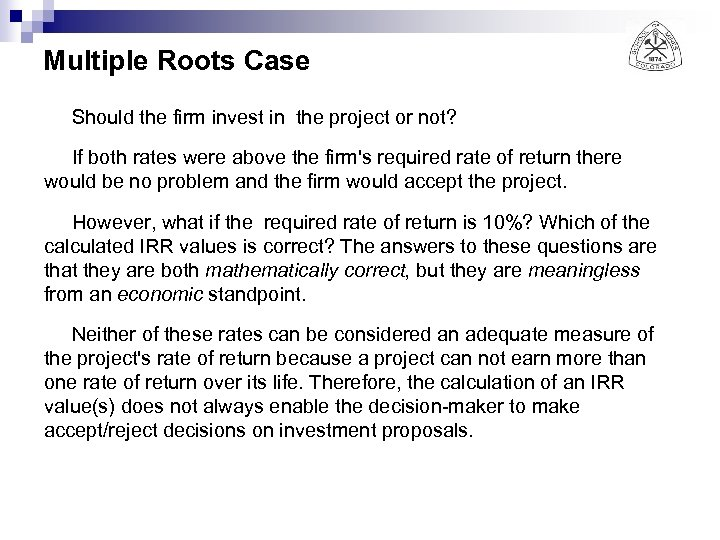Multiple Roots Case Should the firm invest in the project or not? If both rates were above the firm's required rate of return there would be no problem and the firm would accept the project. However, what if the required rate of return is 10%? Which of the calculated IRR values is correct? The answers to these questions are that they are both mathematically correct, but they are meaningless from an economic standpoint. Neither of these rates can be considered an adequate measure of the project's rate of return because a project can not earn more than one rate of return over its life. Therefore, the calculation of an IRR value(s) does not always enable the decision-maker to make accept/reject decisions on investment proposals.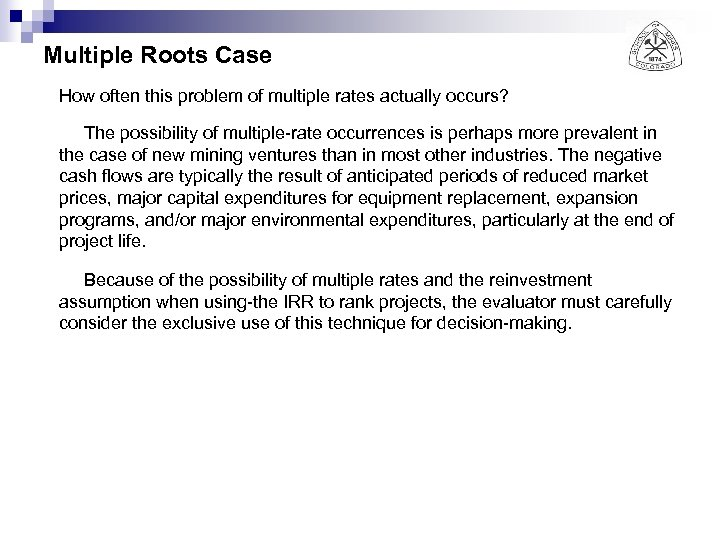Multiple Roots Case How often this problem of multiple rates actually occurs? The possibility of multiple-rate occurrences is perhaps more prevalent in the case of new mining ventures than in most other industries. The negative cash flows are typically the result of anticipated periods of reduced market prices, major capital expenditures for equipment replacement, expansion programs, and/or major environmental expenditures, particularly at the end of project life. Because of the possibility of multiple rates and the reinvestment assumption when using-the IRR to rank projects, the evaluator must carefully consider the exclusive use of this technique for decision-making.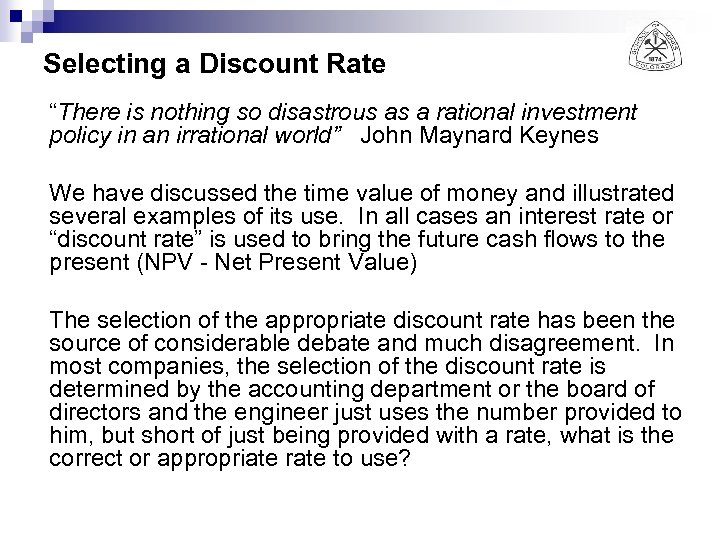Selecting a Discount Rate “There is nothing so disastrous as a rational investment policy in an irrational world” John Maynard Keynes We have discussed the time value of money and illustrated several examples of its use. In all cases an interest rate or “discount rate” is used to bring the future cash flows to the present (NPV - Net Present Value) The selection of the appropriate discount rate has been the source of considerable debate and much disagreement. In most companies, the selection of the discount rate is determined by the accounting department or the board of directors and the engineer just uses the number provided to him, but short of just being provided with a rate, what is the correct or appropriate rate to use?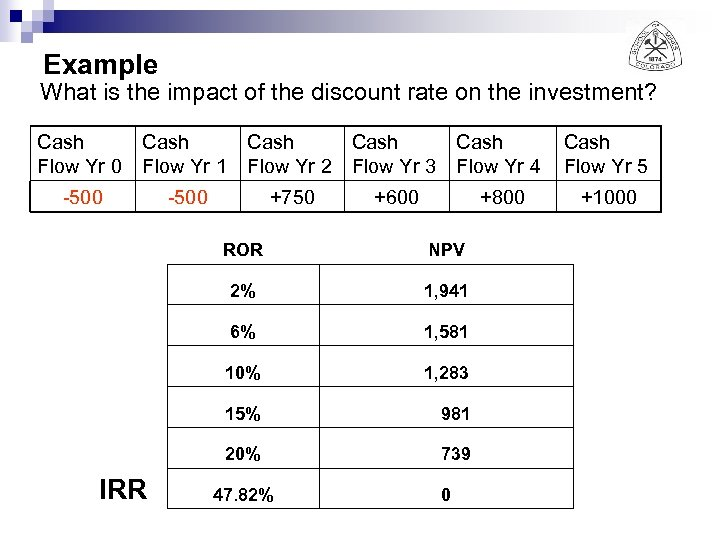Example What is the impact of the discount rate on the investment? Cash Flow Yr 0 Cash Flow Yr 1 -500 Cash Flow Yr 2 Flow Yr 3 -500 +750 Cash Flow Yr 4 Cash Flow Yr 5 +800 +1000 +600 ROR 2% 1, 941 6% 1, 581 10% 1, 283 15% 981 20% IRR NPV 739 47. 82% 0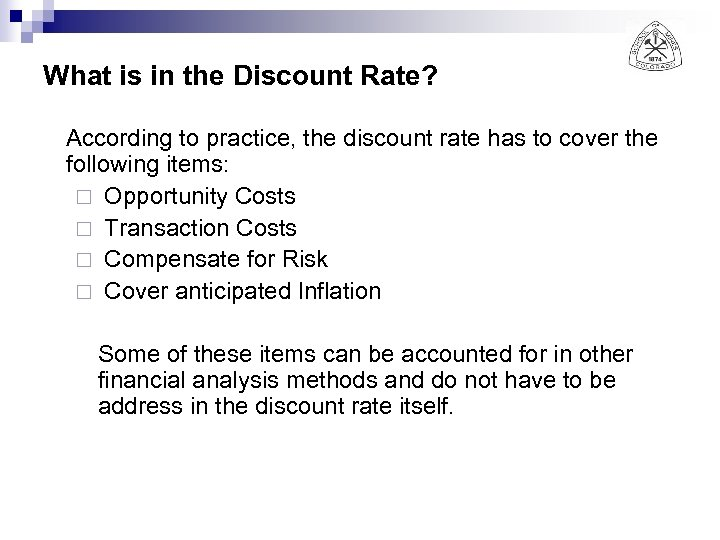What is in the Discount Rate? According to practice, the discount rate has to cover the following items: ¨ Opportunity Costs ¨ Transaction Costs ¨ Compensate for Risk ¨ Cover anticipated Inflation Some of these items can be accounted for in other financial analysis methods and do not have to be address in the discount rate itself.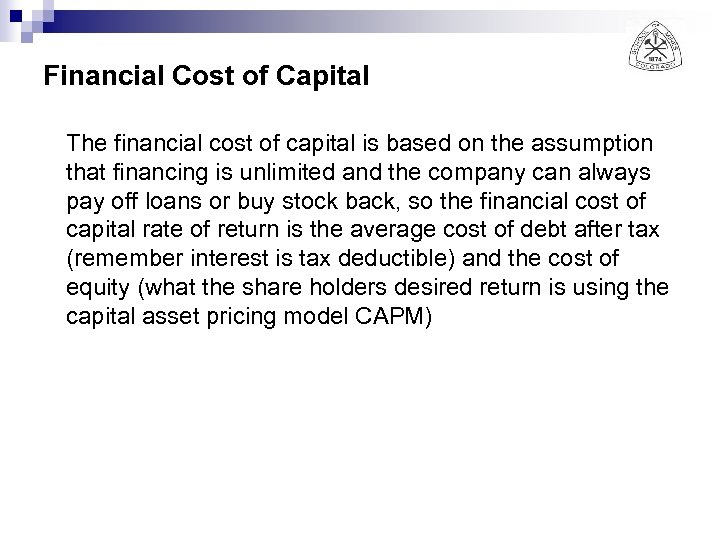Financial Cost of Capital The financial cost of capital is based on the assumption that financing is unlimited and the company can always pay off loans or buy stock back, so the financial cost of capital rate of return is the average cost of debt after tax (remember interest is tax deductible) and the cost of equity (what the share holders desired return is using the capital asset pricing model CAPM)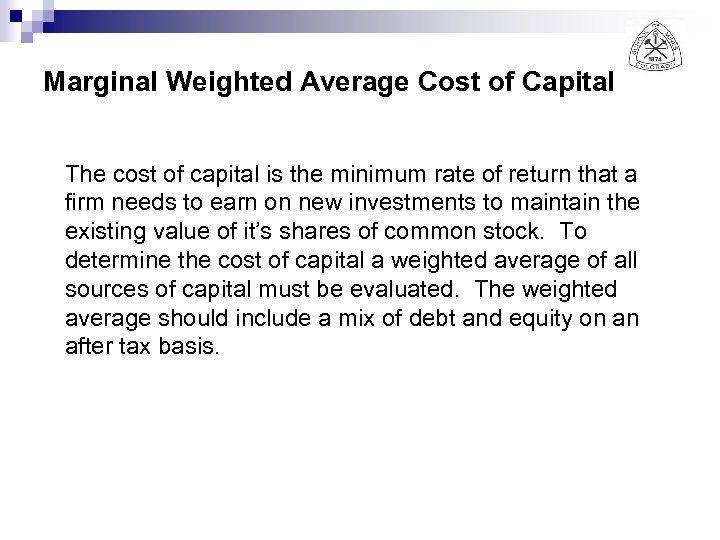Marginal Weighted Average Cost of Capital The cost of capital is the minimum rate of return that a firm needs to earn on new investments to maintain the existing value of it’s shares of common stock. To determine the cost of capital a weighted average of all sources of capital must be evaluated. The weighted average should include a mix of debt and equity on an after tax basis.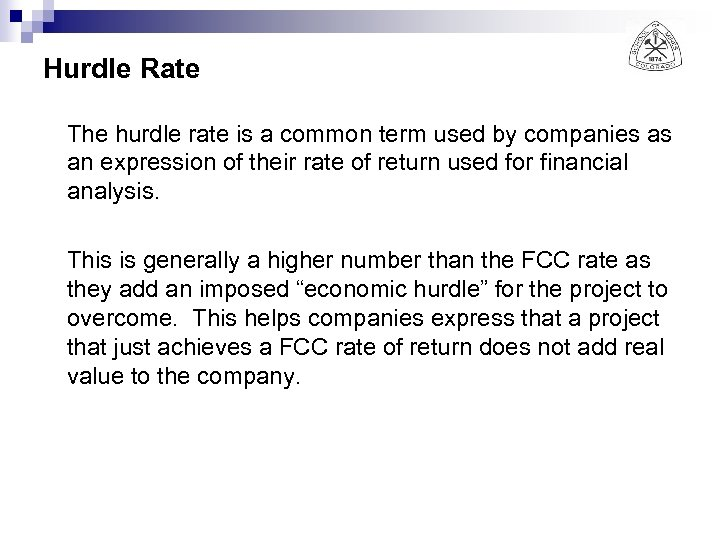Hurdle Rate The hurdle rate is a common term used by companies as an expression of their rate of return used for financial analysis. This is generally a higher number than the FCC rate as they add an imposed “economic hurdle” for the project to overcome. This helps companies express that a project that just achieves a FCC rate of return does not add real value to the company.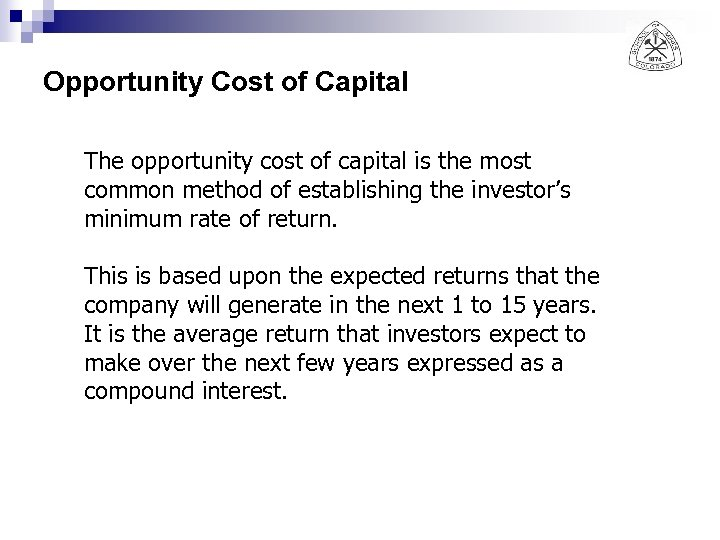Opportunity Cost of Capital The opportunity cost of capital is the most common method of establishing the investor’s minimum rate of return. This is based upon the expected returns that the company will generate in the next 1 to 15 years. It is the average return that investors expect to make over the next few years expressed as a compound interest.